# RD Sharma Solutions For Class 12 Maths Exercise 19.1 Chapter 19 Indefinite Integrals

RD Sharma Solutions for Class 12 Maths Exercise 19.1 Chapter 19 Indefinite Integrals is provided here. In 19.1 of Chapter 19, we shall discuss primitive or antiderivative of indefinite integrals. For all questions enlisted in Class 12 Chapter 19, solutions are solved by BYJU’S experts, based on CBSE guidelines to help students gain competence on the concept.

From the exam perspective, solutions are prepared using shortcut techniques, which speed up students ability to solve problems. Students may keep the textbook aside and use RD Sharma Class 12 Solutions for revision purpose. Also, students can refer and download the PDF from the links provided below.

## Download the PDF of RD Sharma Solutions For Class 12 Chapter 19 – Indefinite Integrals Exercise 19.1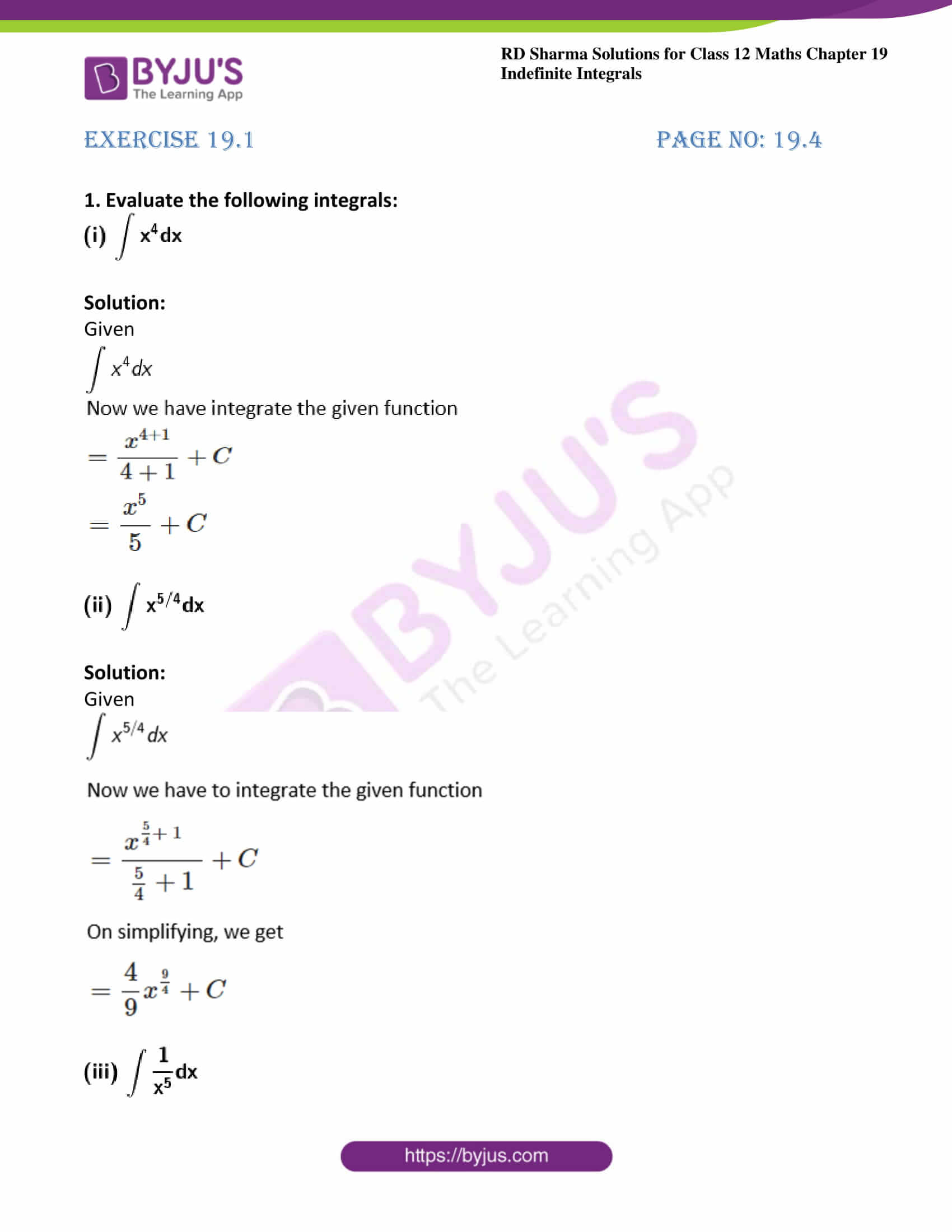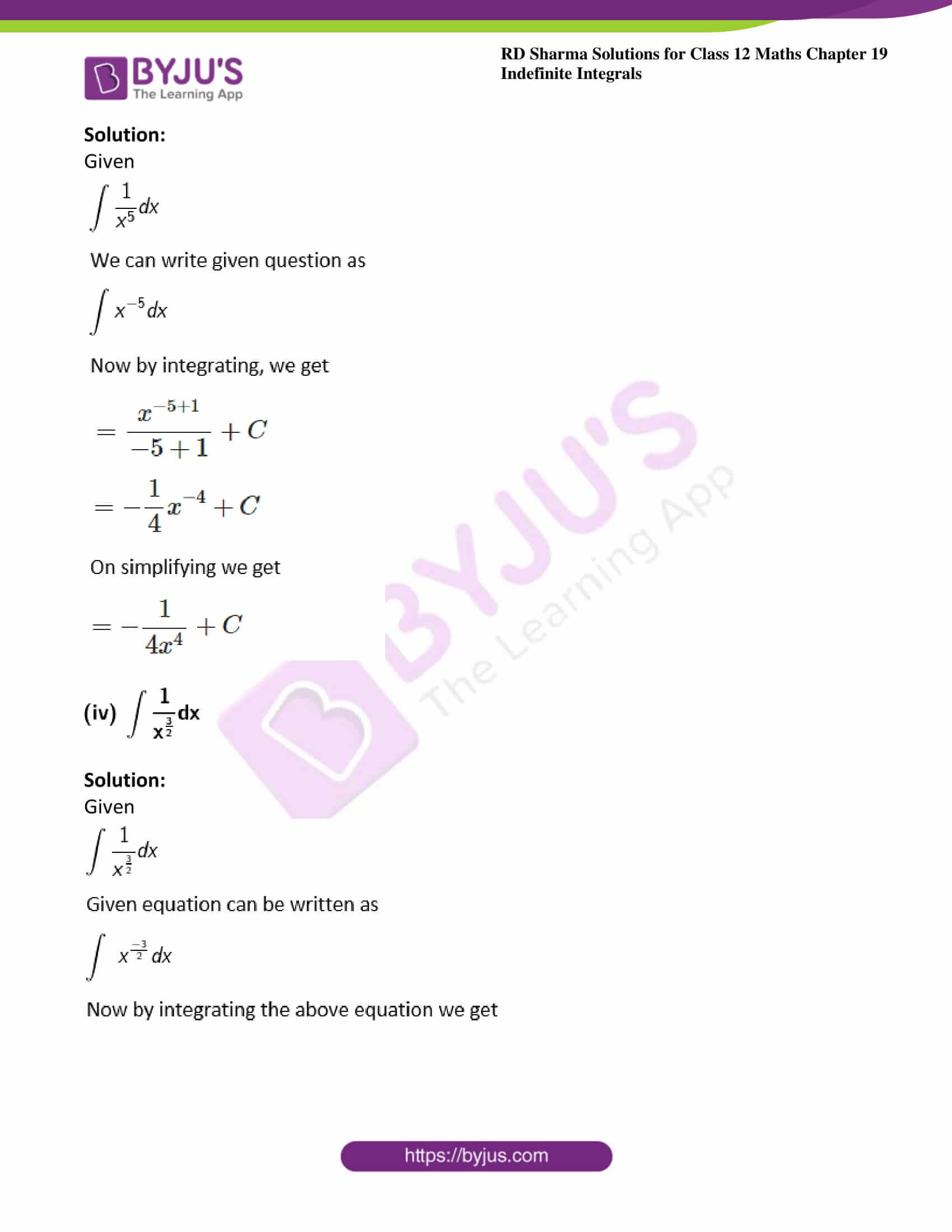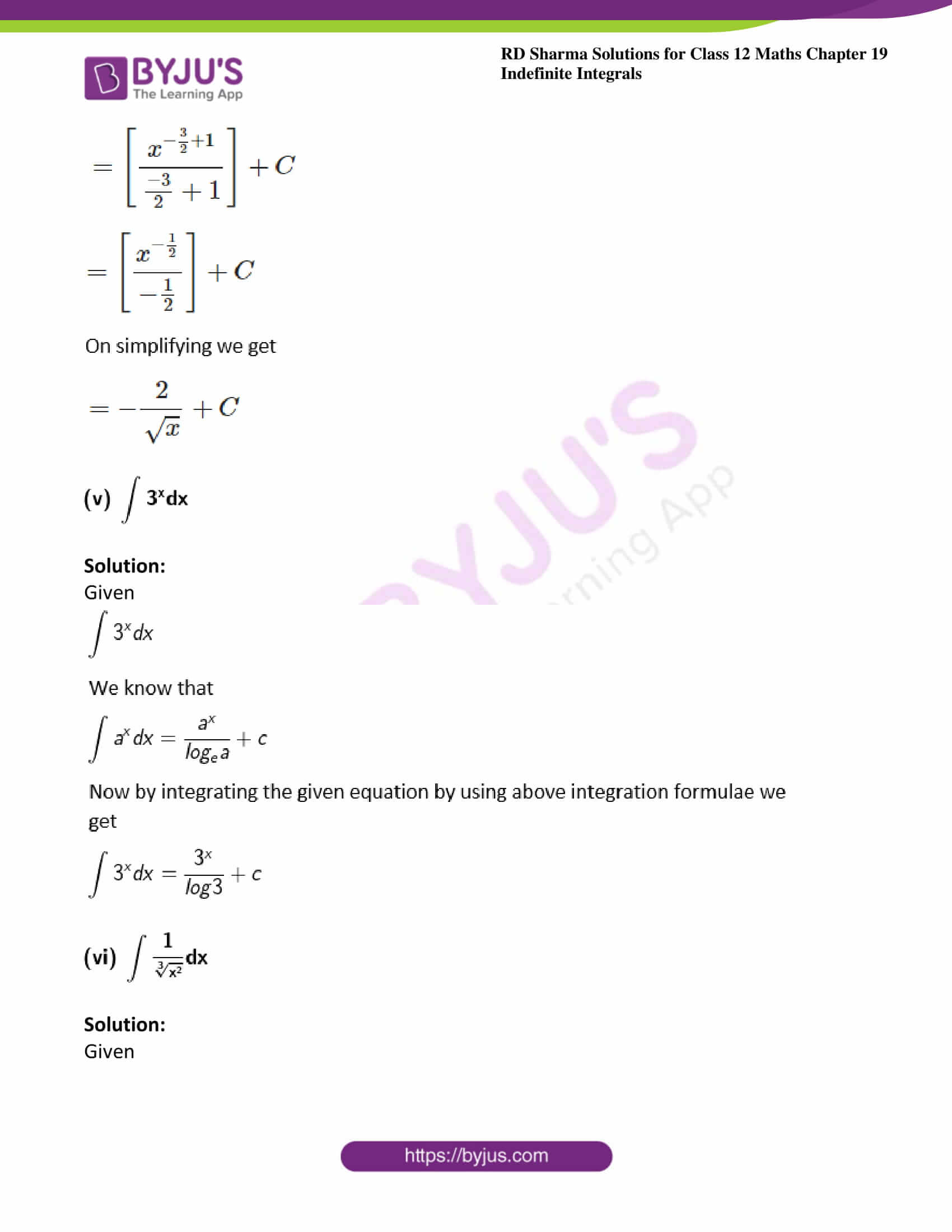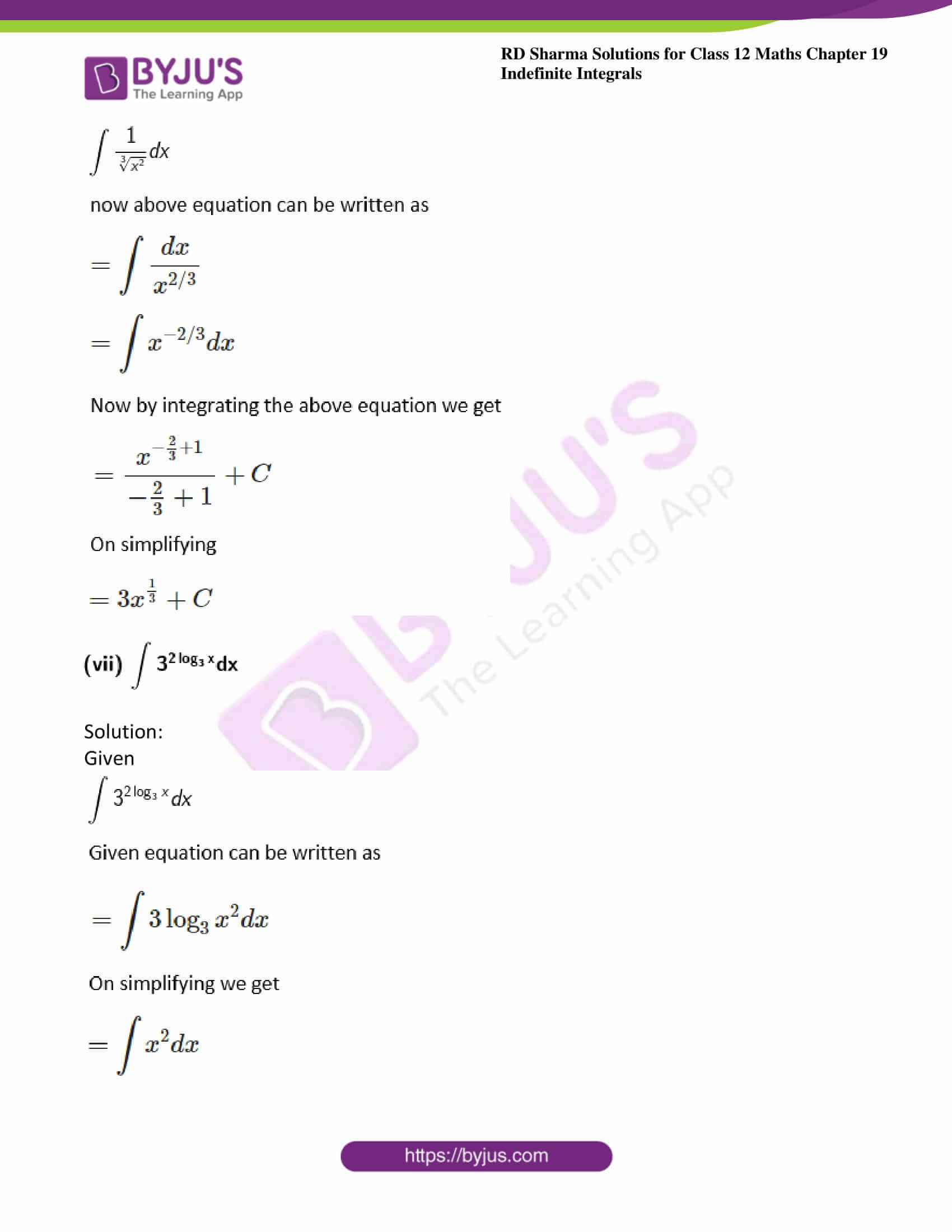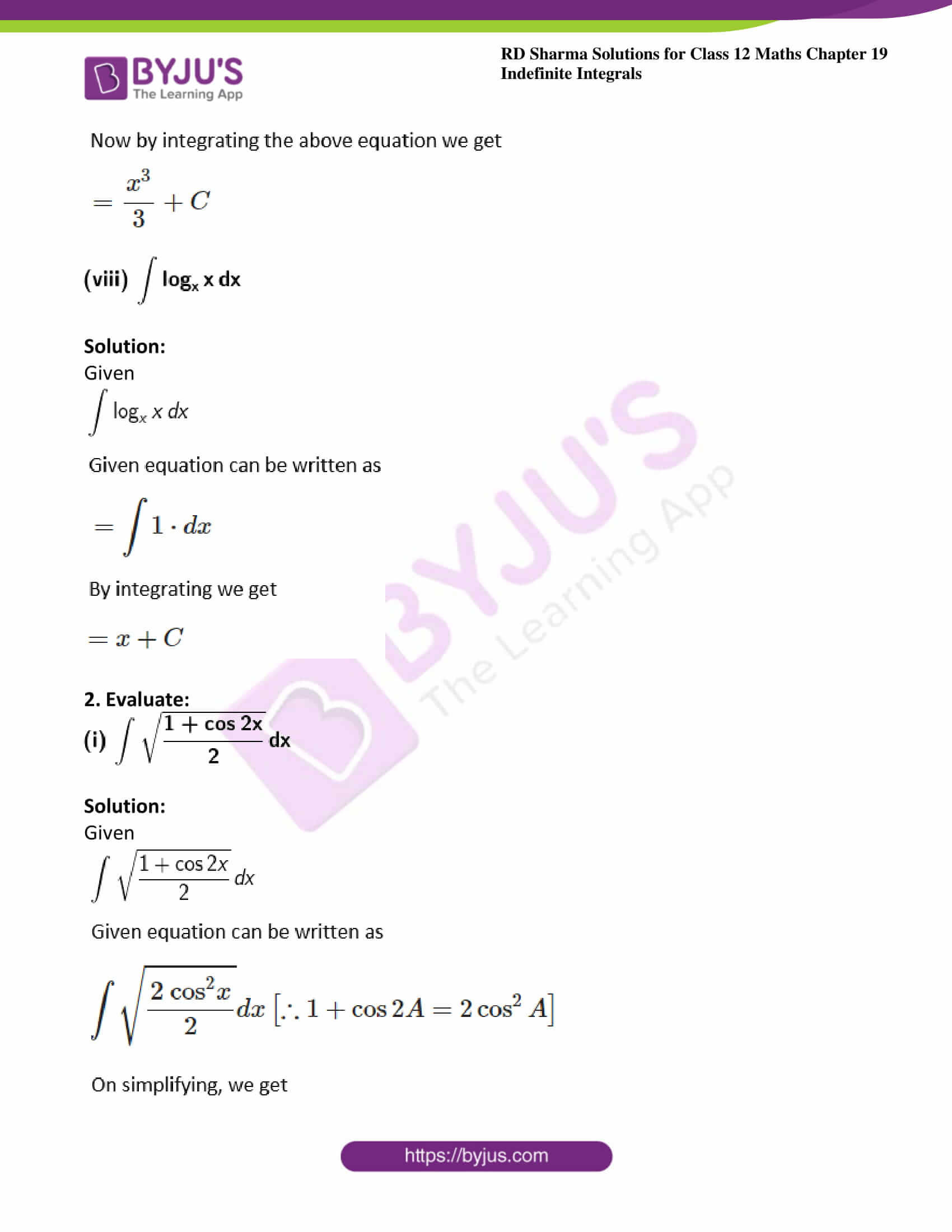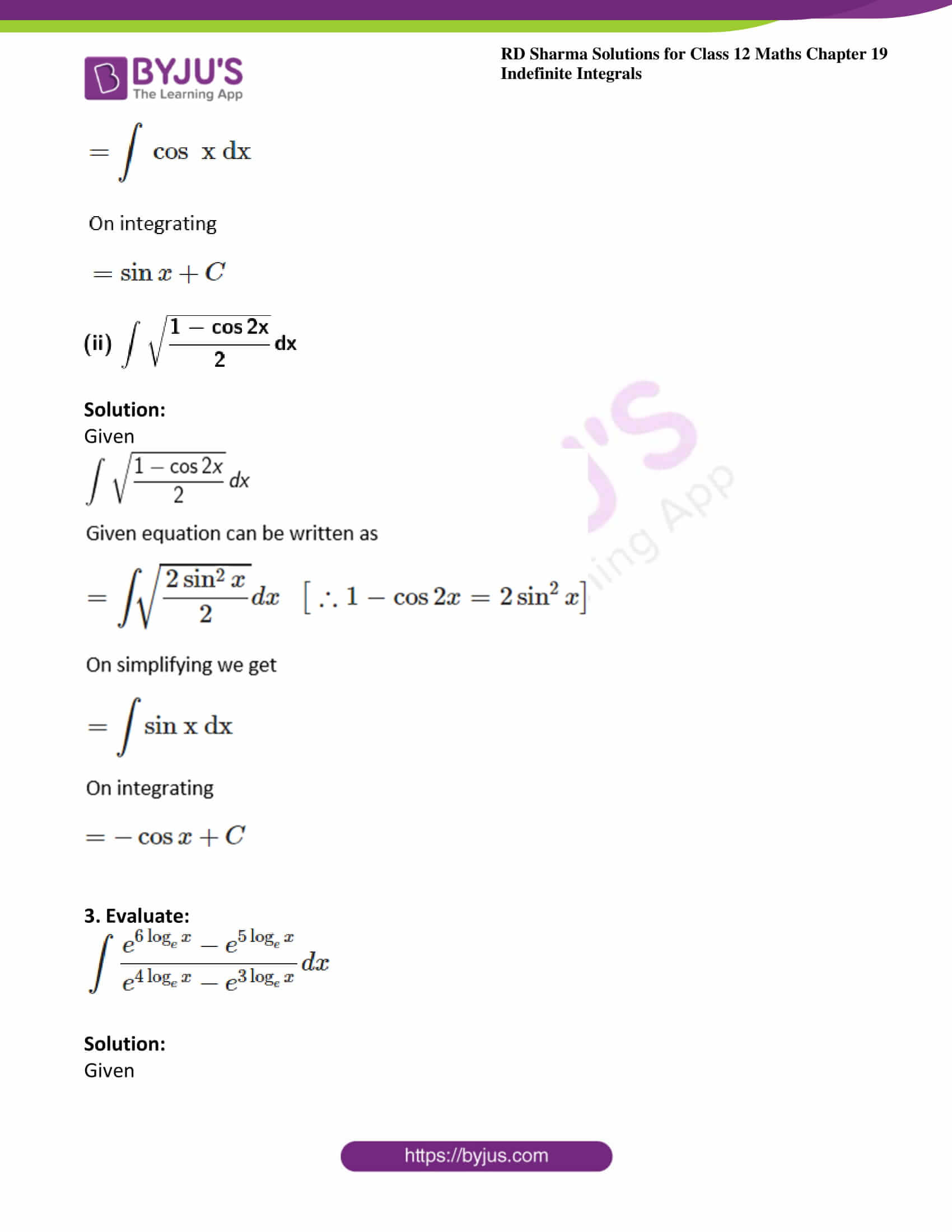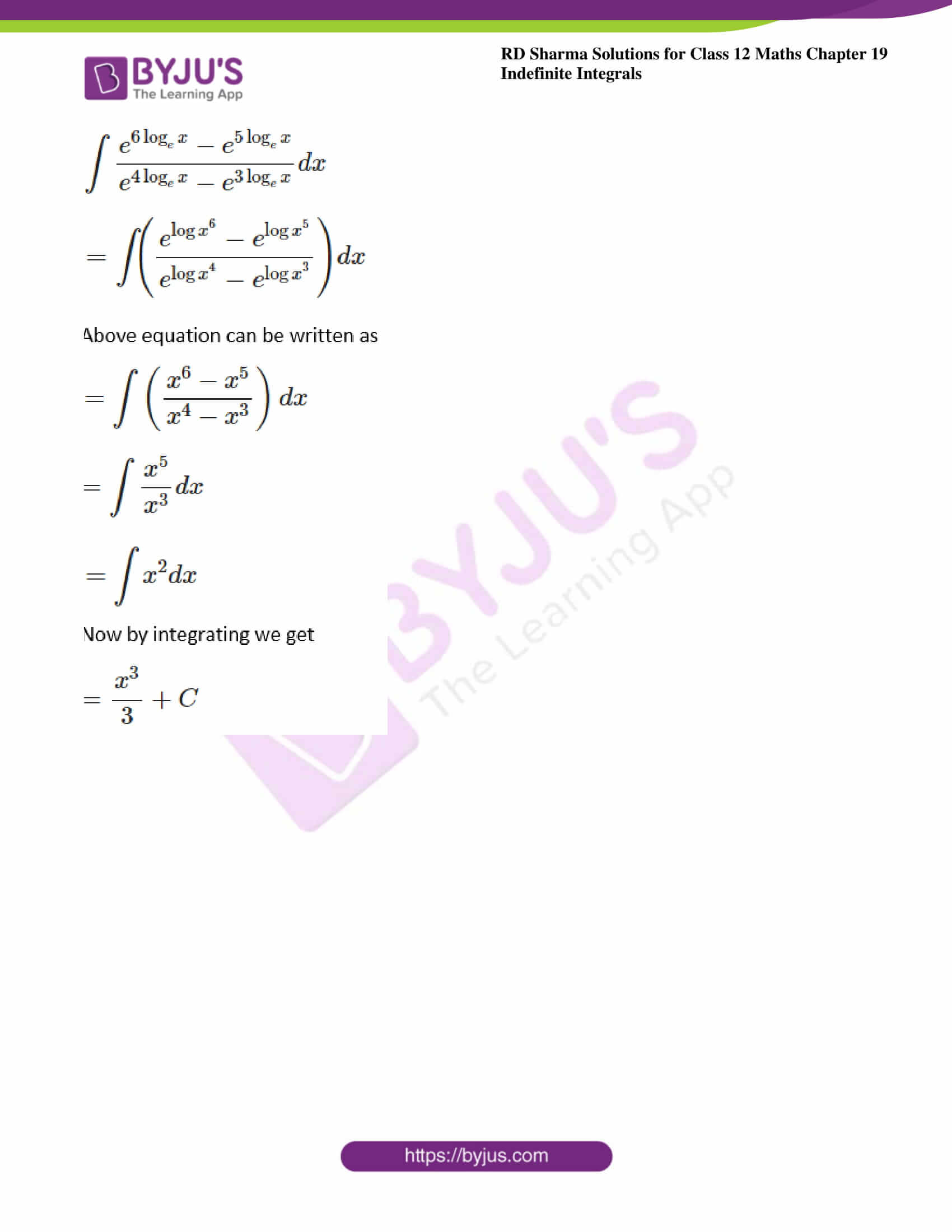### Exercise 19.1 Page No: 19.4

1. Evaluate the following integrals: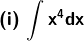Solution:

Given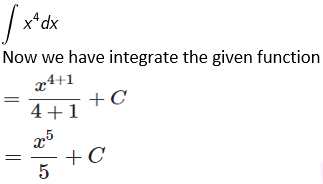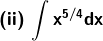Solution:

Given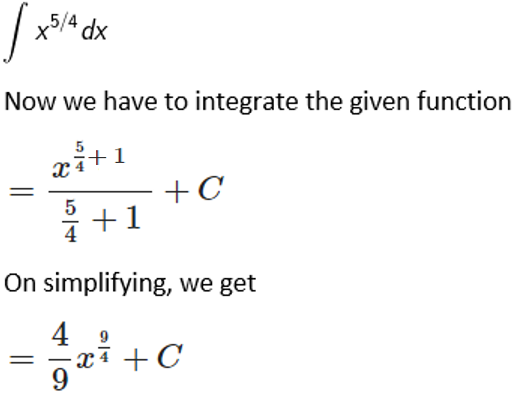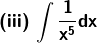Solution:

Given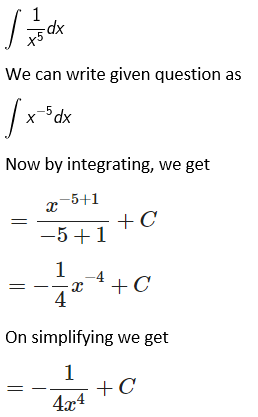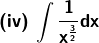Solution:

Given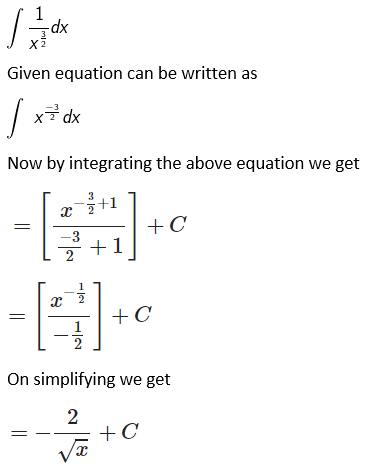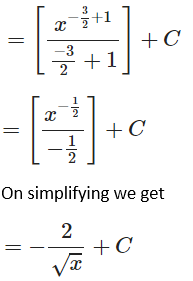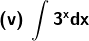Solution:

Given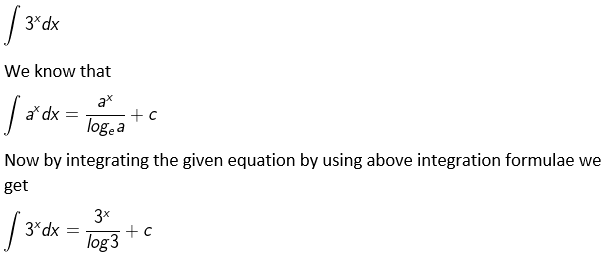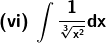Solution:

Given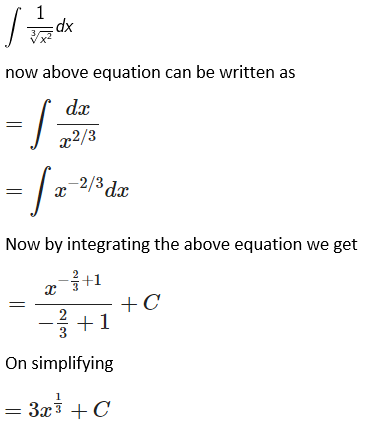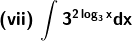Solution:

Given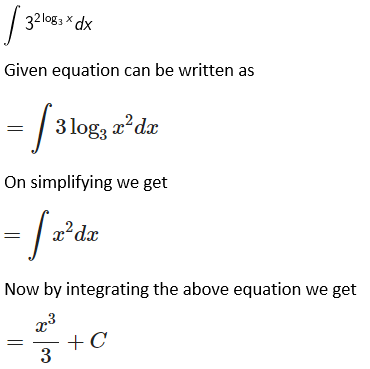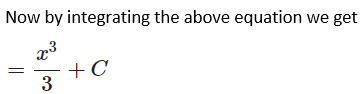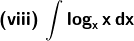Solution:

Given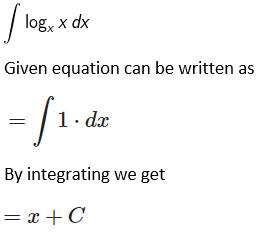2. Evaluate: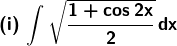Solution:

Given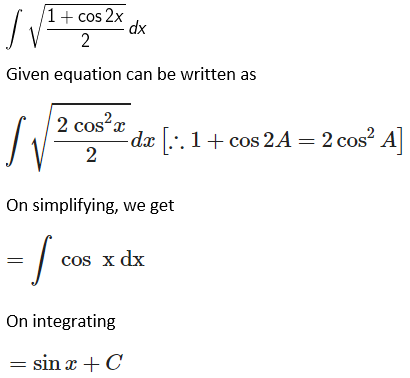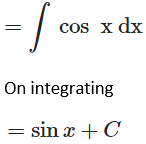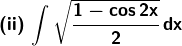Solution:

Given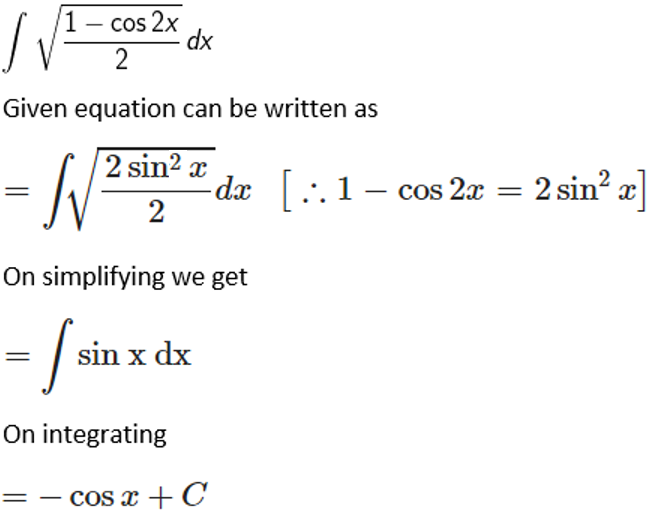3. Evaluate: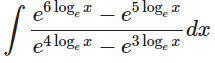Solution:

Given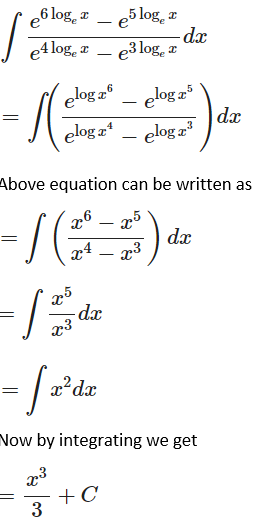### Access other exercises of RD Sharma Solutions For Class 12 Chapter 19 – Indefinite Integrals

Exercise 19.2 Solutions

Exercise 19.3 Solutions

Exercise 19.4 Solutions

Exercise 19.5 Solutions

Exercise 19.6 Solutions

Exercise 19.7 Solutions

Exercise 19.8 Solutions

Exercise 19.9 Solutions

Exercise 19.10 Solutions

Exercise 19.11 Solutions

Exercise 19.12 Solutions

Exercise 19.13 Solutions

Exercise 19.14 Solutions

Exercise 19.15 Solutions

Exercise 19.16 Solutions

Exercise 19.17 Solutions

Exercise 19.18 Solutions

Exercise 19.19 Solutions

Exercise 19.20 Solutions

Exercise 19.21 Solutions

Exercise 19.22 Solutions

Exercise 19.23 Solutions

Exercise 19.24 Solutions

Exercise 19.25 Solutions

Exercise 19.26 Solutions

Exercise 19.27 Solutions

Exercise 19.28 Solutions

Exercise 19.29 Solutions

Exercise 19.30 Solutions

Exercise 19.31 Solutions

Exercise 19.32 Solutions